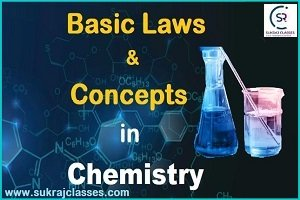# Important law’s and Concepts in Chemistry

Chemistry topic is-“Important law’s and Concepts in Chemistry”, is important for all competitive exams like CET- Common eligibility Test, SSC CGL, SSC CHSL, RRB NTPC, UPSC and other state civil services exams. In these exams, almost 4-5 questions are coming from Chemistry.

## Important law’s and Concepts in Chemistry:-

Law of Conservation of Mass:-

The Law of Conservation of Mass was proposed by “Antoine Lavoisier” in 1789. According to this law, “Matter can neither be created nor destroyed in a chemical reaction”.

• For any chemical change total mass of active reactants are always equal to the mass of the product formed. In other words, the mass of any one element at the beginning of a reaction will equal the mass of that element at the end of the reaction.
• It is a derivation of Dalton’s atomic theory ‘Atoms neither be created nor be destroyed’.

Total masses of reactants = Total masses of products + Masses of unreacted reactants.

• Mass and matter may not be able to be created or destroyed, but can change forms to other substances like liquids, gasses, solids, etc.
• For example: – when wood burns, the mass of the soot, ashes, and gases, equals the original mass of the charcoal and the oxygen when it first reacted. So, the mass of the product equals the mass of the reactant.
• Exception to this law is- nuclear reactions where mass is converted into energy.

This law was discovered in 1811 by “Amedeo Avogadro”.

Avogadro’s Law states that “Equal volumes of gases under identical temperature and pressure will contain equal numbers of particles (atoms, ions, molecules, electrons, etc.)”.

Boyle’s Law:-

Boyle’s law states that “the absolute pressure exerted by a given mass of an ideal gas is inversely proportional to the volume it occupies if the temperature and amount of gas remain unchanged within a closed system”.

• Boyle’s law, also called Mariotte’s law

P (1/V)

P = k/V (where, k = constant, V= volume)

Charles’ Law:-

Charles’s law is an experimental gas law that describes how gases tend to expand when heated.

• It states that “when the pressure on a sample of a dry gas is held constant, the Kelvin temperature and the volume will be in direct proportion”.

V = kT

Dalton’s law of partial pressure:-

Dalton’s law of partial pressures states that the pressure of a mixture of gases is equal to the sum of the partial pressure (that each individual gas) would exert by itself at the same volume and temperature.

Pt P1P2 + P3 …..+ Pn.

Where, Pt is total pressure of the mixture of gases and P1P2P3, … Pn. are the partial pressures of the individual gases.

Henry’s Law:-

Henry’s Law states that at the amount of gas that is dissolved in a liquid is directly proportional to the partial pressure of that gas above the liquid when the temperature is kept constant.

P ∝ C

P = kH .C

where,

• ‘P’ – partial pressure of the gas.
• ‘C’ – concentration of the dissolved gas.
• ‘kH – Henry’s law constant of the gas).

Ideal Gas Law:-

The ideal gas law, also called the general gas equation.

The ideal gas law states that the product of the pressure and the volume of one gram molecule of an ideal gas is equal to the product of the absolute temperature of the gas and the universal gas constant.

PV = nRT

where,

• P is the pressure.
• V is the volume.
• n is the amount of substance.
• R is the ideal gas constant.
• T is the absolute temperature of the gas.

Periodic Law:-

• The periodic law was developed independently by Dmitri Mendeleev and Lothar Meyer in 1869.
• The Periodic Law states that the physical and chemical properties of the elements recur in a systematic and predictable way when the elements are arranged in order of increasing atomic number.

Gay-Lussac’s Law:-

This law states that at given temperature and pressure the volumes of all gaseous reactants and products bear a simple whole number ratio to each other.

Graham’s Laws of Diffusion and Effusion:-

• Graham’s law of effusion was formulated by Scottish physical chemist Thomas Graham in 1848.
• Graham’s Laws states that “the rate of diffusion or effusion of a gas is inversely proportional to the square root of its molecular mass”.

Law of Constant Proportions:-

• This law was proposed by Joseph Proust in 1799.
• This law state that “All pure samples of the same chemical compounds contain the same elements combined in the same proportion by mass”.
• For example: – The composition of CO2 obtained by different means always having same C: O ratio.

Law of Multiple Proportion:-

• This law was proposed by Jhon Dalton in 1804.
• This law states that “When two elements combine to form two or more than two different compounds then the different masses of one element B which combine with fixed mass of the other element bear a simple ratio to one another.”

Law of Reciprocal Proportion:-

• This law was proposed by “Richer” in 1794.
• This law states that ‘when two elements combine separately with third element and form different types of molecules, their combining ratio is directly reciprocated if they combine directly.”

For More:

If you like and think that General Science (Chemistry) topic on “Important law’s and Concepts in Chemistry” was helpful for you, Please comment us. Your comments/suggestions would be greatly appreciated. Thank you to be here. Regards – Team SukRaj Classes.